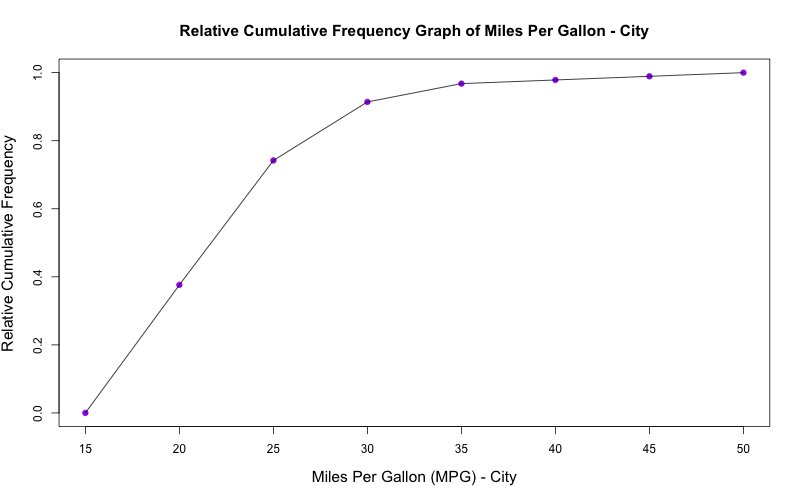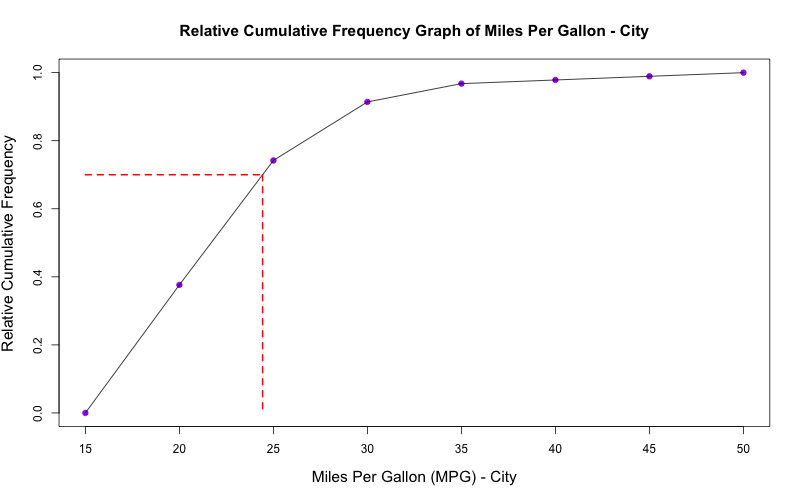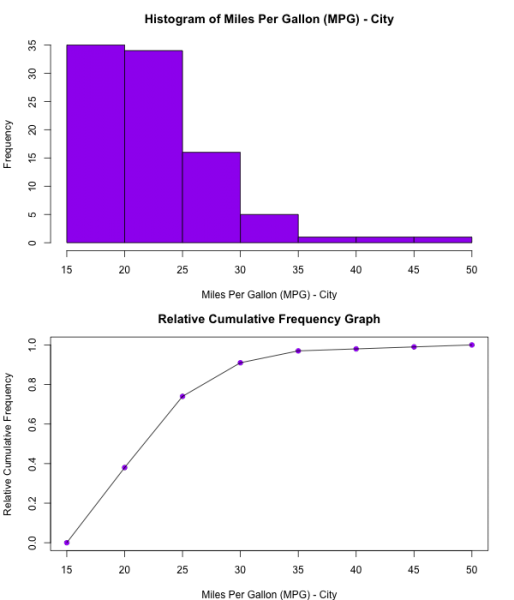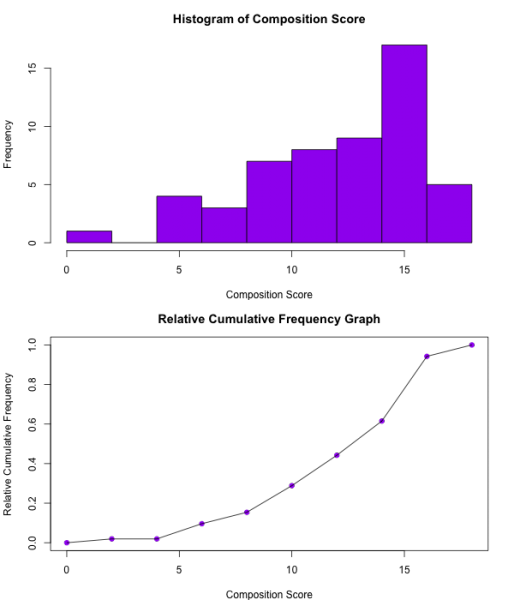Constructing and Interpreting a Relative Cumulative Frequency Graph (Ogive)

Overview
An ogive graph serves as a graphical representation of the cumulative relative frequency distribution for quantitative variables. In other words, these graphs plot the percentile on the y-axis and the quantitative variable on the x-axis. They are interpreted as follows: for example, let's say that the 10th percentile corresponds to an x-value of 20. This would mean that 10% of the data in this given dataset is at or below 20.

Slope: The steeper the slope between two x-values, the higher the frequency of data between those two values. For example, if the slope between x1 and x2 is zero, there are no data points between the two x-values. A very high positive slope between  x1 and x2 indicates that there are a lot of data points between the two x-values.

Point Estimate: Given a percentile of interest, they can be used to estimate the value of your dataset associated with that percentile.

Example - Constructing a Cumulative Frequency Graph (Ogive)
The following data represents Miles Per Gallon (city) estimates for cars selected at random from among 1993 passenger car models that were listed in both the Consumer Reports issue and the PACE Buying Guide. The data was obtained from the Cars93 dataset. With the provided dataset, construct a relative cumulative frequency graph.

25  18  20  19  22  22  19  16  19  16  16  25  25  19  21  18  15  17  17  20  23  20  29  23  22  17  21  18  29  20  31
23  22  22  24  15  21  18  46  30  24  42  24  29  22  26  20  17  18  18  17  18  29  28  26  18  17  20  19  23  19  29
18  29  24  17  21  24  23  18  19  23  31  23  19  19  19  20  28  33  25  23  39  32  25  22  18  25  17  21  18  21  20

1. To simplify subsequent steps, begin by sorting the data from least to greatest:

15  15  16  16  16  17  17  17  17  17  17  17  17  18  18  18  18  18  18  18  18  18  18  18  18  19  19  19  19  19  19
19  19  19  19  20  20  20  20  20  20  20  20  21  21  21  21  21  21  22  22  22  22  22  22  22  23  23  23  23  23  23
23  23  24  24  24  24  24  25  25  25  25  25  25  26  26  28  28  29  29  29  29  29  29  30  31  31  32  33  39  42  46

2. Using the sorted data above, construct the following table:

 Range Frequency Relative Frequency Cumulative Frequency Cumulative Percentile 15 ≤ x < 20 35 0.376 35 0.376 20 ≤ x < 25 34 0.366 69 0.742 25 ≤ x < 30 16 0.172 85 0.914 30 ≤ x < 35 5 0.054 90 0.968 35 ≤ x < 40 1 0.011 91 0.978 40 ≤ x < 45 1 0.011 92 0.989 45 ≤ x < 50 1 0.011 93 1.000

3. Using the range and cumulative percentile data shown above, construct the following graph:Determining Percentiles Using Cumulative Frequency Graphs

Once a relative cumulative frequency graph has been constructed. One can use the graph to calculate percentile values.

Example

For the Cars93 MPG data set, determine the fuel efficiency that corresponds to the 70th percentile.

 Solution: 1. On the y-axis, find the value that corresponds to 0.70.  2. As shown on the right, draw a horizontal line to the right of 0.7. Then draw a vertical line at the point where the horizontal hits the ogive's line segment. 3. Use the vertical line to estimate the value on the x-axis. 4. The 70th percentile for fuel efficiency corresponds to 24 MPG.Comparison Between Histograms and Relative Cumulative Frequency Graphs
There is a direct association between the slope of the Cumulative Frequency Graph for certain intervals and the corresponding frequency count in the same interval of the associated histogram. Notice in Example Two (below) that slope between 2 and 4 is 0, and that the frequency count is also 0 for this section of the histogram. Likewise, between interval 14-16, the slope is greatest in the Relative Cumulative Frequency Graph, while the frequency count during this interval is also the greatest among the other bins.

 GRAPH A GRAPH B